# How to increase the size of an Array

## How to increase the size of an Array?

In this article, I am going to discuss how to increase the size of an array in C and C++. Please read our previous article where we discussed how to create an array in stack and heap memory.

##### How to increase the size of an Array in C and C++?

Let say we have an integer array of size five which is created inside the heap memory. This array is pointed by an integer pointer p and the spaces having some elements. For better understanding, please have a look at the below image.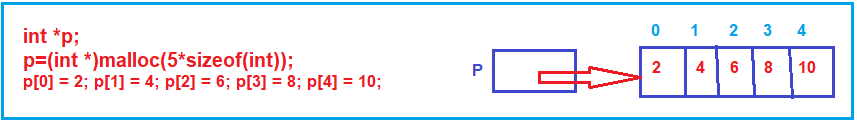Now, we want to add few more elements to the array i.e. the size 5 is not sufficient for me and I want a larger array. One method for increasing the size of an array is, take one more pointer (let’s called q) and create a new array with the required large size (let say size 10). So, the pointer q is now pointing to an array with size 10 as shown in the below image.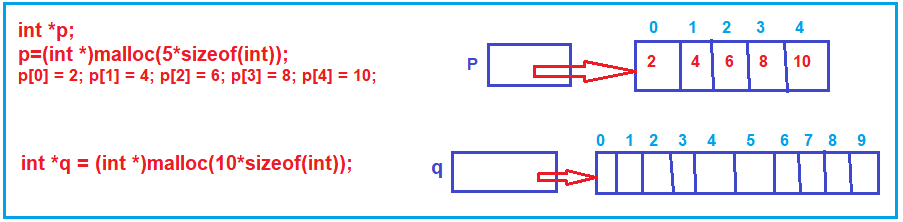We cannot increase the size of the same array. So, alternatives we are creating a bigger size array. Now transfer all the elements from the smaller array (i.e. p) to the bigger array (i.e. q). Here, we are using a for loop to copy the elements from p to q as shown in the below image.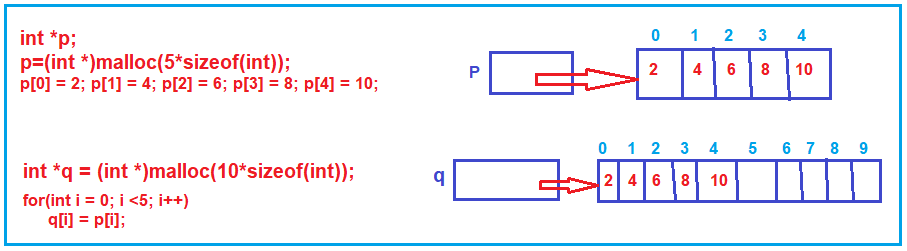We want to increase the size of p, actually, p is a pointer and it points to an array. Now we have to make the pointer p pointing to the larger array. But before making the pointer point to the larger array, first, let us delete the smaller array. If you don’t delete the unused memory, then it may cause a memory leak issue. To delete the array, call the function free bypassing the pointer p as an argument as follows:

free(p);

Then make pointer p point on q i.e. p =q. So wherever q is pointing, p will also point there. Now new location where p is pointing is the larger array as shown in the below image.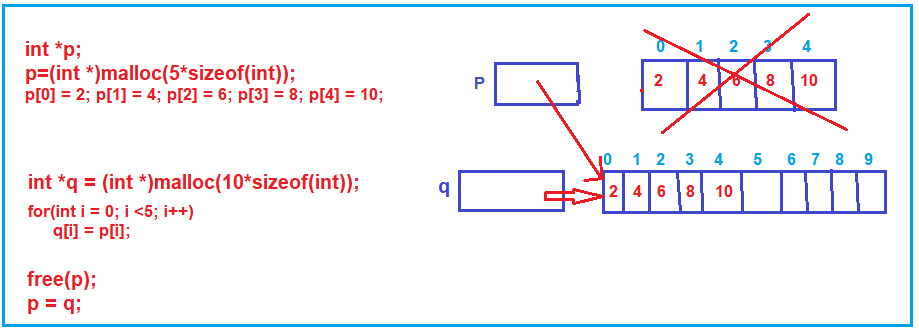Now the larger array is pointed by both p and q. Let us remove q from thereby making q=null; so, q will no more pointing to the larger array as q is null. If we say p then it is pointing to the larger array as shown in the below image.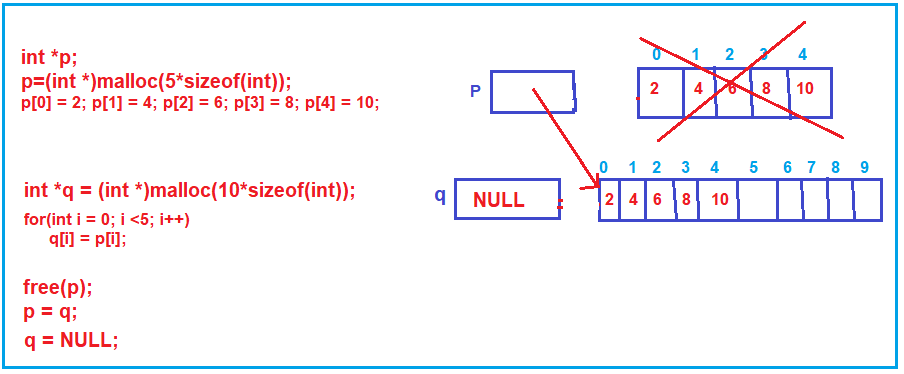##### Increasing the Array size in C Language:

The following example demonstrates how to increase the array size in the C language. Here, we are using the malloc function to create the array in the heap memory and the free function to remove the unused memory.

```#include <stdio.h>
#include <stdlib.h>
int main ()
{
int *p, *q;
p = (int *) malloc (5 * sizeof (int));
p = 2;
p = 4;
p = 6;
p = 8;
p = 10;
q = (int *) malloc (10 * sizeof (int));

for (int i = 0; i < 5; i++)
{
q[i] = p[i];
}

free (p);
p = q;
q = NULL;

for (int i = 0; i < 5; i++)
{
printf("%d ", p[i]);
}
return 0;
}```

Output: 2 4 6 8 10

##### Increasing the Array size in C++ Language:

The following example demonstrates how to increase the array size in the C++ language. Here, we are using the new operator to create the array in the heap memory and delete to remove the unused memory.

```#include <iostream>
using namespace std;
int main()
{
int *p = new int;
p = 2;
p = 4;
p = 6;
p = 8;
p = 10;
int *q = new int;;

for (int i = 0; i < 5; i++)
{
q[i] = p[i];
}

delete []p;
p = q;
q = NULL;

for (int i = 0; i < 5; i++)
{
cout << p[i];
}
return 0;
}```
##### Why the array size cannot be grown?

Because the memory for the array should be contiguous. Let say, initially we created an array whose starting address is 1000 and end address is 1020 i.e. contiguous from 1000 to 1020. Later after some point in time, we want to increase the size. But it is not guaranteed the next location onwards i.e. 1021 is freely available. It might be possible that those next memory locations are occupied by some other objects in the program. If the consecutive locations are not free then the array cannot be increased. That is the reason we say that array size cannot be increased.

In the next article, I am going to discuss 2-Dimensional Arrays in C and C++ with Examples. Here, in this article, I try to explain How to increase the size of an array in C and C++ and I hope you enjoy this How to increase the size of an array article.